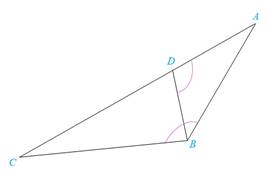Chapter 5.3, Problem 40EElementary Geometry For College St...

7th Edition
Alexander + 2 others
ISBN: 9781337614085

Solutions

Chapter
SectionElementary Geometry For College St...

7th Edition
Alexander + 2 others
ISBN: 9781337614085
Textbook Problem

In the figure, ∠ A B C ≅ ∠ A D B . Find AB if A D = 2 and D C = 6 .To determine

To find:

The length of the side AB if the given sides are AD = 2, DC = 6 and ABCADB.

Explanation

Definition:

AA:

If two angles of one triangle are congruent to two angles of another triangle, then the triangles are similar.

CSSTP:

Corresponding sides of similar triangles are proportional.

Description:

The given figure is shown below.

Figure 1

From the given figure, it is observed that there are two triangles ABC and ADB.

Now draw the two triangles separately as shown below.

Figure 2

Figure 3

Given that ABCADB and the point A is common for both the triangles. So by identity AA.

From the above mentioned AA definition, the two triangles ABC and ADB are similar since the two angles of one triangle are congruent to two angles of another triangle. Hence, ΔABCΔABD.

From the definition of CSSTP, corresponding sides of similar triangles are proportional.

It is given that AD = 2, DC = 6

Still sussing out bartleby?

Check out a sample textbook solution.

See a sample solution

The Solution to Your Study Problems

Bartleby provides explanations to thousands of textbook problems written by our experts, many with advanced degrees!

Get Started

x+1x1=5

Applied Calculus for the Managerial, Life, and Social Sciences: A Brief Approach

Proof Prove that sinasinbab for all a and b.

Calculus: Early Transcendental Functions (MindTap Course List)

For y = x sin x, y = _____. a) x cos x b) x cos x + 1 c) cos x d) x cos x + sin x

Study Guide for Stewart's Single Variable Calculus: Early Transcendentals, 8th